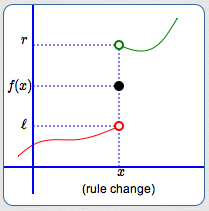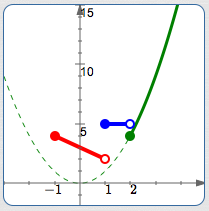﻿ Piecewise-Defined Functions

#Piecewise-Defined Functions

A piecewise-defined function has different rules for different inputs.

Here is a typical piecewise-defined function:

$$f(x) = \cases { -x + 3 &\text{if }\quad -1 \le x \lt 1\cr 5 &\text{if }\quad 1 \le x \lt 2\cr x^2 &\text{if }\quad x\ge 2 }$$

Or, with slightly different formatting and notation:

$$f(x) = \cases { -x + 3\,, &\text{if }\quad x\in [-1,1)\cr 5\,, &\text{if }\quad x\in [1,2)\cr x^2\,, &\text{if }\quad x\in [2,\infty) }$$

Recall that ‘$\,x\in [-1,1)\,$’ means that $\,x\,$ is a member of the interval $\,[-1,1)\,.$

## Finding Function Values for a Piecewise-Defined Function

This function $\,f\,$ uses three rules (one for each row). That is, it has three ‘pieces’ (hence the name piecewise-defined function):

• For inputs in the interval $\,[-1,1)\,,$ the linear function $\,-x+3\,$ is used for the outputs.
• For inputs in the interval $\,[1,2)\,,$ the constant function $\,5\,$ is used for the outputs.
• For inputs in the interval $\,[2,\infty)\,,$ the quadratic function $\,x^2\,$ is used for the outputs.

Here are some examples:

 Find this function value: $f(-1)$ Which interval is $\,x\,$ in? $-1\in [-1,1)$ So, use this rule ... $-x+3\,$  (first row) Solution: $f(-1) = -(-1) + 3 = 4$
 Find this function value: $f(0)$ Which interval is $\,x\,$ in? $0\in [-1,1)$ So, use this rule ... $-x+3\,$  (first row) Solution: $f(0) = -(0) + 3 = 3$
 Find this function value: $f(0.9)$ Which interval is $\,x\,$ in? $0.9\in[-1,1)$ So, use this rule ... $-x+3\,$  (first row) Solution: $f(0.9) = -(0.9) + 3 = 2.1$
 Find this function value: $f(1)$ Which interval is $\,x\,$ in? $1\in [1,2)\,$ So, use this rule ... $5\,$  (second row) Solution: $f(1) = 5$
 Find this function value: $f(1.99999)$ Which interval is $\,x\,$ in? $1.99999 \in [1,2)$ So, use this rule ... $5\,$  (second row) Solution: $f(1.99999) = 5$
 Find this function value: $f(2)$ Which interval is $\,x\,$ in? $2 \in [2,\infty)$ So, use this rule ... $x^2\,$  (third row) Solution: $f(2) = 2^2 = 4$
 Find this function value: $f(\pi)$ Which interval is $\,x\,$ in? $\pi \in [2,\infty)$ So, use this rule ... $x^2\,$  (third row) Solution: $f(\pi) = \pi^2$

## Reading a Piecewise-Defined Function Aloud

If forced to read the function $$\cssId{s51}{ f(x) = \cases { -x + 3 &\text{if }\quad -1 \le x \lt 1\cr 5 &\text{if }\quad 1 \le x \lt 2\cr x^2 &\text{if }\quad x\ge 2 }}$$ aloud, it is typically read from left-to-right and top-to-bottom.

One possible reading could go like this:

Eff of ex equals negative ex plus three, if ex is greater than or equal to negative one and less than one;

it equals five, if ex is greater than or equal to one and less than two;

it equals ex squared, if ex is greater than or equal to two.

More often, though, people working with such a formula will just look at it and use it!

Here are additional things you should be able to do with a piecewise-defined function:

Finally, you should be aware of variations in notation for piecewise-defined functions.

## Finding the Domain of a Piecewise-Defined Function

The domain is easy to get from the formula alone. Just ‘put together’ (union) the input sets from all the rows. Combined, these are the values of $\,x\,$ that $\,f\,$ knows how to act on.

$$f(x) = \cases { -x + 3 &\text{if }\quad -1 \le x \lt 1\cr 5 &\text{if }\quad 1 \le x \lt 2\cr x^2 &\text{if }\quad x\ge 2 }$$
 top row $[-1,1)$second row $\,[1,2)\,$third row $\,[2,\infty)\,$union, to get the domain of $\,f\,$ $\text{dom}(f) = [-1,\infty)\,$By the way, the range of $\,f\,$ is nowhere near as easy to get from the formula. Once you have a graph, though, the range is easy to obtain: just use the ‘collapse the graph into the $\,y\,$-axis’ method, as described in this earlier lesson.

## Graphing a Piecewise-Defined Function

When a graph is ‘pieced together’ using different rules, as in a piecewise-defined function, you may need up to three ‘dots’ to fully understand what's happening at a place where rules are changing. This is illustrated in the sketch below:In this example, three rules are needed to fully understand what happens at and near $\,x\,$:

• There is a rule that applies at $\,x\,.$ This produces the solid (filled-in) dot, with coordinates $\,(x,f(x))\,.$ This is an actual point on the graph of the function; it is an (input,output) pair.
• There is a rule that applies to the left of $\,x\,.$ The hollow dot at $\,\color{red}{(x,\ell)}\,$ is not a point on the graph; it is not an (input,output) pair.

When the input is $\,x\,,$ the output is not $\,\ell\,$! Instead, this hollow dot is used to show limiting behavior. It answers the question: What happens to the outputs as the inputs approach $\,x\,$ from the left-hand side? (Answer: the outputs approach the number $\,\ell\,$)

• There is a rule that applies to the right of $\,x\,.$ The hollow dot at $\,\color{green}{(x,r)}\,$ is not a point on the graph; it is not an (input,output) pair.

When the input is $\,x\,,$ the output is not $\,r\,$! Instead, this hollow dot is used to show limiting behavior. It answers the question: What happens to the outputs as the inputs approach $\,x\,$ from the right-hand side? (Answer: the outputs approach the number $\,r\,$)

Remember: for a given value of $\,x\,,$ there can be at most one solid dot. To be a function, each allowable input must have exactly one output.

Next, we graph:

$$\cssId{s105}{f(x) = \cases { -x + 3 &\text{if }\quad -1 \le x \lt 1\cr 5 &\text{if }\quad 1 \le x \lt 2\cr x^2 &\text{if }\quad x\ge 2 }}$$

The ‘pieces’ may be graphed in any order. Here, the choice is made to move from left-to-right on the number line (from top-to-bottom in the formula).On $\color{red}{[-1,1)}$, $\,f\,$ is linear, since $\,\color{red}{-x+3}\,$ is of the form $\,mx + b\,.$

The endpoint $\,-1\,$ is included in the interval, indicated by the use of the bracket ‘$\,[\,$’ in interval notation.

The endpoint $\,1\,$ is excluded from the interval, indicated by the use of the parenthesis ‘$\,)\,$’ in interval notation.

 $x$ is ... $-1$ Included or excluded? included Substitute into $\,-x+3\,$: $-(-1) + 3 = 1 + 3 = 4$ Plot the point ... $(-1,4)$ ... using this kind of dot: solid
 $x$ is ... $1$ Included or excluded? excluded Substitute into $\,-x+3\,$: $-(1) + 3 = -1 + 3 = 2$ Plot the point ... $(1,2)$ ... using this kind of dot: hollow

Important: the point $\,(1,2)\,$ is not a point on the graph of $\,f\,.$ This hollow dot is used to show limiting behavior: as the inputs approach $\,1\,$ from the left-hand side, the outputs approach $\,2\,.$On $\,\color{blue}{[1,2)}\,,$ $\,f\,$ is constant with value $\,\color{blue}{5}\,,$ so it graphs as a horizontal segment.

 $x$ is ... $1$ Included or excluded? included Substitute into the constant function $\,5\,$: $5$ Plot the point ... $(1,5)$ ... using this kind of dot: solid
 $x$ is ... $2$ Included or excluded? excluded Substitute into the constant function $\,5\,$: $5$ Plot the point ... $(2,5)$ ... using this kind of dot: hollow

Important: the point $\,(2,5)\,$ is not a point on the graph of $\,f\,.$ This hollow dot is used to show limiting behavior: as the inputs approach $\,2\,$ from the left-hand side, the outputs approach $\,5\,.$On $\,\color{green}{[2,\infty)}\,,$ $\,f\,$ is quadratic, since $\,\color{green}{x^2}\,$ is of the form $\,ax^2 + bx + c\,.$ Thus, it graphs as a piece of a parabola on this interval.

Think about what the entire graph of $\,y = x^2\,$ looks like (it is lightly dashed here), and draw in the portion for the inputs $[2,\infty)\,.$

 $x$ is ... $2$ Included or excluded? included Substitute into $\,x^2\,$: $2^2 = 4$ Plot the point ... $(2,4)$ ... using this kind of dot: solid

Here is the description of the piecewise-defined function $\,f\,,$ color-coded with its graph (above):

$$\cssId{s159}{f(x) = \cases { \color{red}{-x + 3} &\text{if }\quad -1 \le x < 1\cr \color{blue}{5} &\text{if }\quad 1 \le x < 2\cr \color{green}{x^2} &\text{if }\quad x\ge 2 }}$$

## Getting the Formula for a Piecewise-Defined Function from its Graph

To go from a graph to a formula, you need additional information for any non-linear and non-constant sections, perhaps like this:There will be three rows in the piecewise-defined function, since there are three ‘pieces’.

You may want to put the proper inputs in place first: left-to-right on the number line corresponds to top-to-bottom in the rows. Be very careful about solid dots and hollow dots!

Put any ‘easy’ formulas in place at the same time (like constant functions and labeled pieces):

$$\cssId{s169}{f(x) = \cases { ... &\text{if }\quad -1 \le x \lt 1\cr 5 &\text{if }\quad 1 \le x \lt 2\cr x^2 &\text{if }\quad x\ge 2 }}$$

It remains only to get the formula for the linear section:

• Read off the coordinates $\,(-1,4)\,$ and $\,(1,2)$
• Find the slope: $$\cssId{s173}{m = \frac{2-4}{1 - (-1)} = \frac{-2}{2} = -1}$$
• Use point-slope form with either endpoint: $$\cssId{s175}{y - 2 = -(x - 1)}$$
• Solve for $\,y\,$:

\begin{align} y &= -(x-1) + 2\cr &= -x+1+2\cr &= -x+3 \end{align}

Put the formula $\,-x+3\,$ in the top row. Done!

## Variations in Notation for Piecewise-Defined Functions

There are a variety of styles for formatting piecewise-defined functions, including (but not limited to):

• Using the word ‘for’ instead of ‘ if ’ to specify the input values
• Putting commas after each rule
• Putting commas at the very end of all rows but the last

Lots of different combinations are possible. Here are some examples:

$$\cssId{s187}{ f(x) = \cases { -x + 3\color{red}{\,,} &\text{if }\quad -1 \le x \lt 1\cr 5\color{red}{\,,} &\text{if }\quad 1 \le x \lt 2\cr x^2\color{red}{\,,} &\text{if }\quad x\ge 2 }}$$

(Commas after each rule)

$$\cssId{s189}{f(x) = \cases { -x + 3\,, &\text{if }\quad -1 \le x \lt 1\color{green}{\,,}\cr 5\,, &\text{if }\quad 1 \le x \lt 2\color{green}{\,,}\cr x^2\,, &\text{if }\quad x\ge 2 }}$$

(Commas at the very end of all rows but the last)

$$\cssId{s191}{f(x) = \cases { -x + 3\,, &\text{ }\style{color:blue}{\text{for}}\quad -1 \le x \lt 1\cr 5\,, &\text{ }\style{color:blue}{\text{for}}\quad 1 \le x \lt 2\cr x^2\,, &\text{ }\style{color:blue}{\text{for}}\quad x\ge 2 }}$$

(Use the word ‘for’ instead of ‘if’)

With beautiful typesetting (including all the words ‘ if ’ lined up vertically), this author prefers the simplest formatting, with no extra punctuation.